# MCAT Physical : Electric Potential Energy

## Example Questions

### Example Question #11 : Electrostatics And Electrical Fields

If the electrical potential energy between two equal charges quadruples, describe the change in the distance between the particles.

The distance was not changed

The distance was halved

The distance was quartered

The distance was doubled

The distance was quartered

Explanation:

Electrical potential energy is given by the equation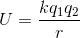.

Electrical potential energy is inversely proportional to the distance between the two charges. If the energy is quadrupled, then(the distance between the two equal charges) must have decreased proportionally.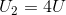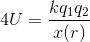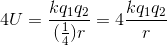### Example Question #12 : Electrostatics And Electrical Fields

For the following: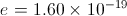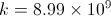If a charge of magnitude +4e is being held in place 3 nm from a charge of -5e which is also being held in place. What is the potential energy of the system?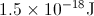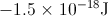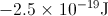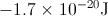Explanation: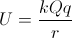Given the equation and plugging in the values of e and k, we get that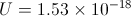It is important to keep in mind that the charge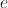is given in the question and must be incorporated into the formula by multiplying each charge by that value.

### Example Question #13 : Electrostatics And Electrical Fields

Which of the following is not true regarding electric potential?

All of these are true statements

The positive terminal of a battery has higher electric potential than the negative terminal

When a positive charge moves from a region of low potential to high potential, the electric field does positive work on the charge

A negative charge moving from low potential to high potential will accelerate

Electric potential can be expressed with units of Volts or Joules per Coulomb

When a positive charge moves from a region of low potential to high potential, the electric field does positive work on the charge

Explanation:

A positive test charge will naturally move from high potential to low potential. If it is moved in the opposite direction, then the electric field will do work against its motion (negative work). This be seen from the equation for electric field work: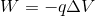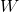is the work done by the electric field,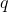is the charge, and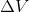is the potential difference. Ifis positive (the final potential is higher than the initial potential) andis also positive, then work done by the field is negative.

### Example Question #14 : Electrostatics And Electrical Fields

How much work is required to bring together the three given charges from infinity to the corners of an equilateral triangle of side length 1cm?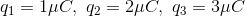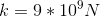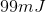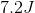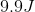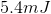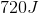Explanation:

Relevant equations:Step 1: Since the work done to assemble the charges equals their potential energy in this arrangment, find the potential energy between each pair of charges. Work is equal to change in potential energy; since the charges start at infinite distance, initially potential energy is equal to zero.

Charges 1 and 2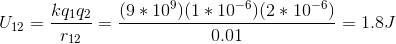Charges 1 and 3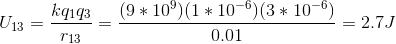Charges 2 and 3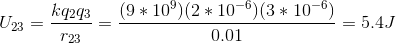Step 2: Add together all these potential energies to find the total energy of the arrangement.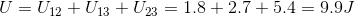### Example Question #15 : Electrostatics And Electrical Fields

A standard AC outlet is capable of delivering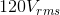of current. What is the maximum possible voltage given by the outlet.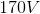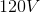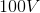Since the given voltage is the root mean squared voltage we must multiply the voltage byto find the maximum voltage.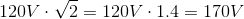We determine that the maximum voltage delivered by the outlet is.